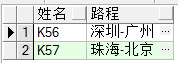###### double7y

2015-07-10 03:57 阅读 3.4k

# plsql多表联系查询的问题

K56 SZ GZ
K57 ZH BJ

SZ 深圳
GZ 广州
ZH 珠海
BJ 北京

K56 深圳-广州
K57 珠海-北京

• 点赞
• 写回答
• 关注问题
• 收藏
• 复制链接分享

#### 5条回答默认 最新

• 已采纳
`````` select a.车次 as 姓名 ,a.城市中文||'-'||b.城市中文 as 路程 from (
select 表A.车次,表B.城市中文 from 表A inner join 表B on 表A.出发城市 = 表B.二字码) a
left join
(select 表A.车次,表B.城市中文 from 表A inner join 表B on 表A.目的城市 = 表B.二字码) b
on a.车次 = b.车次
``````点赞 5 评论 复制链接分享
• ``````select 车次,F || '-' || T FROM (
select 车次,(select 城市中文 from 表B where 表B.城市二字码=出发城市) F
,(select 城市中文 from 表B where 表B.城市二字码=目的城市) T FROM 表A
)
``````
点赞 1 评论 复制链接分享
• select a.cc,b1.name,b2.name from A a left join B b1 on b1.cs=a.cfcs left join B b2 on b2.cs=a.mdcs

点赞 评论 复制链接分享
• 我还以为是什么高深的问题呢？ 这是简单的字段合并 || ,

点赞 评论 复制链接分享
• select x.车次 as 车次,concat(x.城市中文,'-',y.城市中文) as 始末站
from
(select a.车次,b.出发城市中文 from 表A a join 表B b where a.出发城市=b.城市) x
left join
(select a.车次,b.结束城市中文 from 表A a join 表B b where a.结束城市=b.城市) y
on x.车次 = y.车次;
还是楼一牛呀。

点赞 评论 复制链接分享